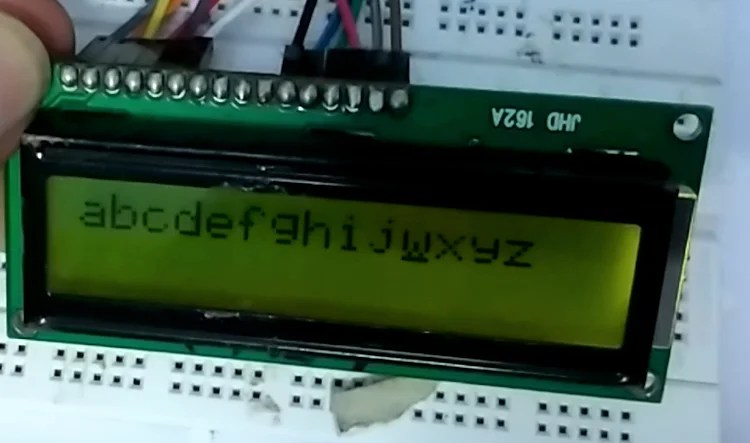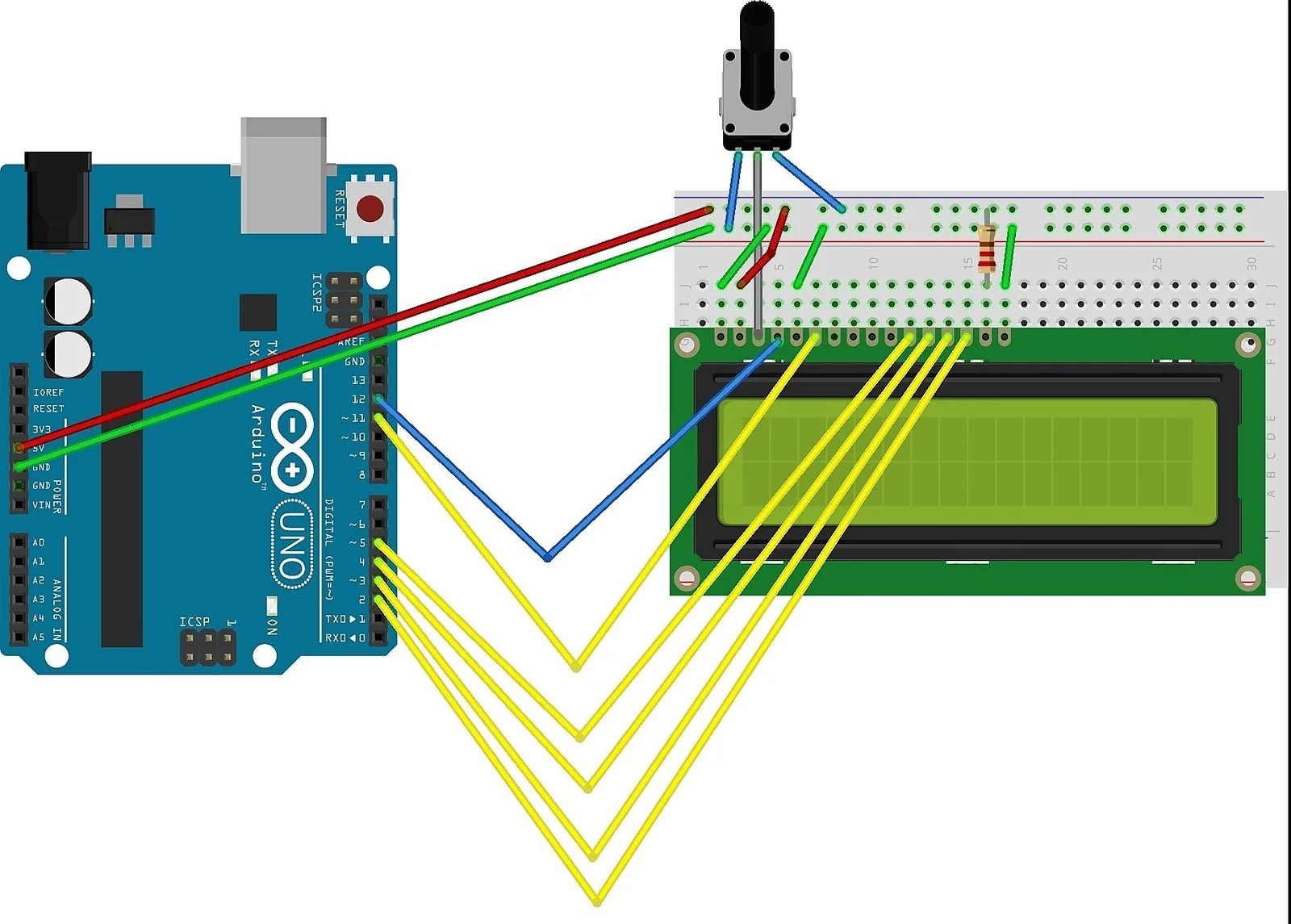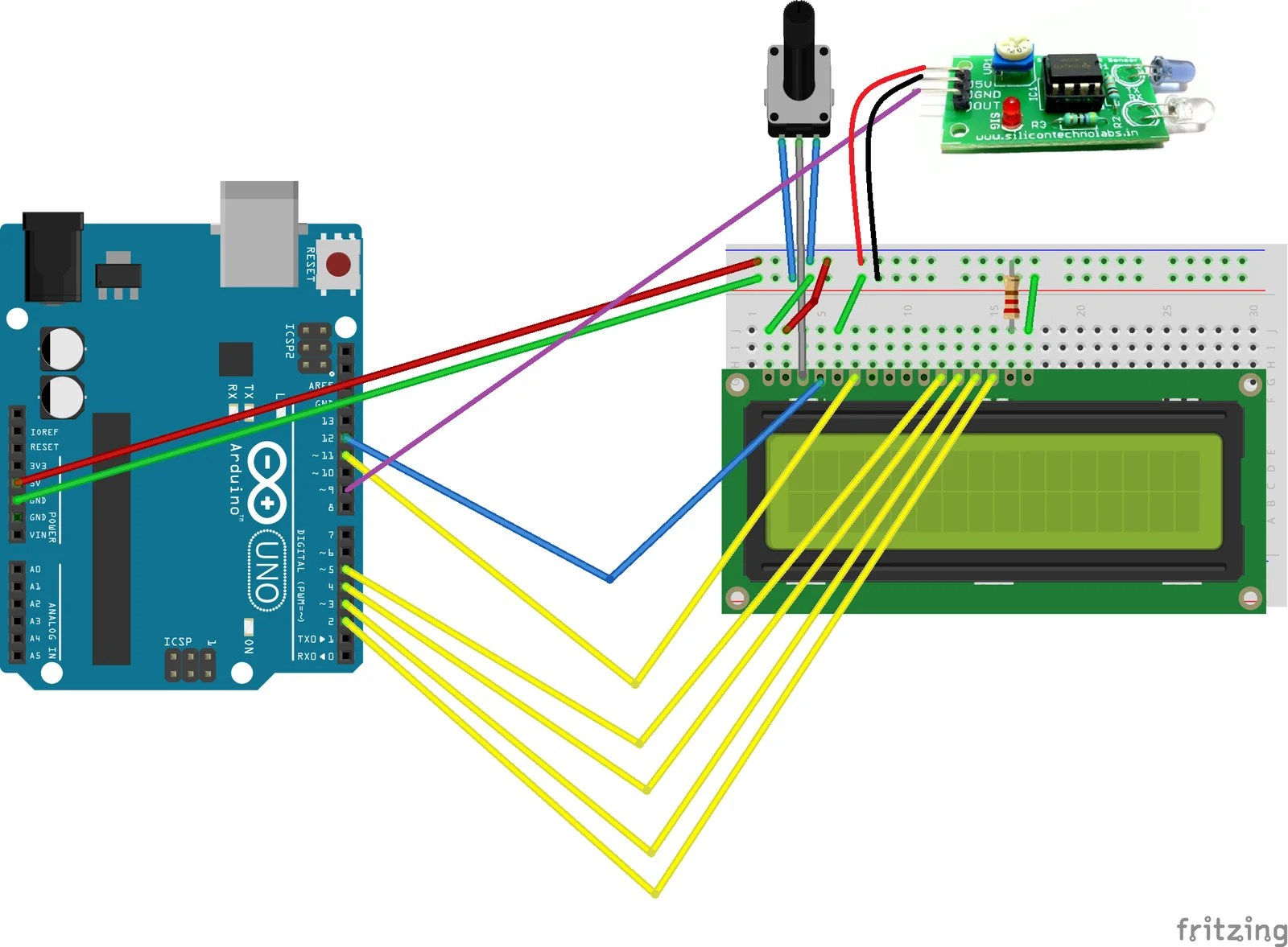# INTERFACING LCD DISPLAY WITH ARDUINO

In this section, we will first learn how to print Alphabets and then output from an IR sensor on LCD display.

BY ASHISH BARTWAL

INTRODUCTION

There are many commands in Arduino through which we can control the output of an LCD display.These commands enable us to move, blink a specific text printed on LCD display. We can code Arduino in such a way that it reads the input from sensors like infrared sensor and then print it on LCD display. Some basic codes that will be required for performing above functions are listed in this project.

LIST OF COMPONENTS REQUIRED

1. Arduino
2. 1602 LCD Screen (16 x 2 LCD)
3. Jumper wires
4. 220ῼ resistor
5. 10k potentiometer
7. Infrared sensor

CIRCUIT DIAGRAM :FIG. Interfacing LCD with ArduinoFIG. Printing IR sensor o/p on LCD

CONNECTION

The circuit:

1. LCD RS pin to digital pin 12
2. LCD Enable pin to digital pin 11
3. LCD D4 pin to digital pin 5
4. LCD D5 pin to digital pin 4
5. LCD D6 pin to digital pin 3
6. LCD D7 pin to digital pin 2
7. LCD R/W pin to ground
8. 220 ῼ resistor: LCD pin 15 and ground
9. Potentiometer wiper to LCD VO pin (pin 3) and ends to +5v and ground of Arduino kit
10. LCD pin 16 to ground
11. Infrared module VCC to +5V
12. Infrared module Gnd to 0V
13. Infrared module DO to digital pin 9 of Arduino
14. Infrared module AO pin will not be used.

FUNCTION OF LCD PINS

Numbering of the pins is done from the viewer’s left-hand side

1. Gnd– This is the ground pin of the LCD display.

1. Vcc– This is the power pin of the LCD display.

1. V– This is the variable voltage pin of the LCD. Brightness of  LCD can be

Adjusted using this pin with a potentiometer.

1. RS– This is the register select pin of the LCD. LCD has two registers, they are-    A)Command Register- It controls the way that data is displayed. This is set as 0 i.e. ground pin. B)Data Register- It controls the content that has to be displayed. This is set as 1 i.e. +5v pin.
2. R/W– This is read or write pin of the LCD.  A) Read operation- Reads data from Arduino. This is set to 0 to perform read operation.B)Write operation-Writes the data as given by external devices such as keypads. This is set to 1 to perform a write operation.
1. E– This is enable pin of the LCD. It enables the LCD to accept data either as a falling edge triggered pin or rising edge triggered pin.

NOTE: Following pins make LCD to work in either 8bit mode (DB0- DB7) or 4bit mode DB4-DB7)

1. DB7-
2. DB6-
3. DB5-
4. DB4-
5. DB3-
6. DB2-
7. DB1-
8. DB0-

COMMANDS USED :

1. LiquidCrystal LCD(12, 11, 5, 4, 3, 2);

Initializes library with the specified interface pins.

1. #include <LiquidCrystal.h>

This is the library code of liquid crystal in Arduino. It is preinstalled in Arduino IDE.

1. begin(16, 2);

It sets the 16 columns and 2 rows in LCD.

1. setCursor(x, y);

Sets the LCD cursor to x row and n coulomb.

1. print(“ ”);

Prints the desired data in the LCD.

1. autoscroll();

1. noAutoscroll();

1. rightToLeft();

Go right for the next character.

1. home();

Sets cursor to its initial position i.e.[ 0,0]

1. clear();

Clear all the available character.

1. scrollDisplayLeft();

Scroll text to one position left.

1. scrollDisplayRight();

Scrolls text to one position right

CODE TO DISPLAY AND CONTROL THE TEXT ON LCD:

**extensive comments are written for a better understanding of code.

AIM: Arduino starts printing the alphabets from ‘a’. Once ‘m’ is printed, remaining alphabets are printed from right to left direction one after the other. And finally when ‘s’ is printed, direction get reversed and characters are printed up to ‘z’.

#include <LiquidCrystal.h>

LiquidCrystal LCD(12, 11, 5, 4, 3, 2); //RS,EN,D4,D5,D6,D7 pin of LCD respectively

int thisChar = ‘a’;                //defines this character ‘a’

void setup()        //in this function we initialize the device connected to Arduino

{

LCD.begin(16, 2);     // set up the LCD’s number of columns and rows

LCD.cursor();       //turn on the  cursor

}

void loop()

{

if (thisChar == ‘m’)               //alphabets till ‘m’ is reached according to alphabetical order

{

LCD.rightToLeft();      //alphabets will move from right to left one after the other when ‘m’ is reached

}

if (thisChar == ‘s’)

{

LCD.leftToRight();      //alphabets will movefrom left to right once ‘s’is reached

}

if (thisChar > ‘z’)

{

LCD.home();       //if character is greater than z than set cursor at thisChar = ‘a’;(0,0) and print ‘a’

}

LCD.write(thisChar);      //print the characters on LCD

delay(1000);

thisChar++;

}

CODE TO PRINT OUTPUT FROM IR SENSOR ON LCD

AIM: Arduino prints value 0 when there is no object in front of IR transmitter and value 1 when there is an obstacle in front.

#include <LiquidCrystal.h>

#define sensor 9      //defines ir sensor connected to digital pin 9 of Arduino

int val=0;        //sets the initial value equal to 0

LiquidCrystal LCD(12, 11, 5, 4, 3, 2);

void setup()

{

pinMode(sensor,INPUT);    //sets sensor i.e.digital pin 9 as input to the Arduino

Serial.begin(9600);

LCD.begin(16, 2);

LCD.print(“OUTPUT IS “);

}

void loop() {

LCD.setCursor(11,0);

Serial.print(val);      //LCD prints val i.e. sensor readings

Serial.print(“val”);

if(val==HIGH)     //if sensor detects object than it glows red i.e.“HIGH”state

{

LCD.print(“1”);     //prints 1 if above condition is satisfied

delayMicroseconds(100);

}

else

{

LCD.print(“0”);     //prints 0 if above condition not satisfied

delayMicroseconds(100);

}

}

NOTE: Hence Arduino prints value 0 when there is no object in front of IR transmitter and value 1 when there is an obstacle in front.

VIDEO TUTORIAL:

Watch this video to see above codes in action:

## One comment

1.Final Grade Calculator says: